# Defining Vectors

• B
metastable
Source: https://mathinsight.org/vectors_cartesian_coordinates_2d_3d
"A vector in three-dimensional space. A representation of a vector a=(a1,a2,a3)a=(a1,a2,a3) in the three-dimensional Cartesian coordinate system."

My question is does representation of a vector to arbitrary precision require 3 values? Can I represent any vector to arbitrary precision with a single value by superimposing a spiral from pole to pole on the surface of a sphere, defining north pole as 0 and south pole as 1, and referencing a point on the spiral as a percentage value. To achieve arbitrary precision I increase the number of "orbits" the superimposed spiral completes around the sphere on its path from north to south. The vector is a ray originating at the center of the sphere, crossing the surface at the referenced point on the spiral. Has this been done before and is it valid?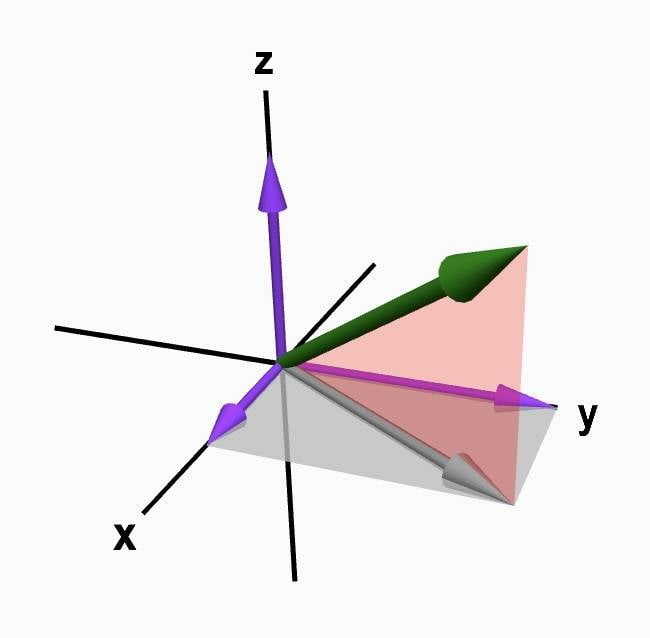Gold Member
2022 Award
Can I represent any vector to arbitrary precision with a single value by superimposing a spiral from pole to pole on the surface of a sphere, defining north pole as 0 and south pole as 1, and referencing a point on the spiral as a percentage value.
Probably so, but it would be a LOT more complicated than just specifying the 3 values since the percent is the trivial part ... you have to specify the spiral's diameter and so forth. Seems very messy. Also it would be horrendous in terms of understandability. You would look at the spiral equation and the percent and have no clue where the end point is.

Homework Helper
single value by superimposing a spiral from pole to pole on the surface of a sphere
You'd still have to provide the radius of the sphere...
Can I represent any vector to arbitrary precision
and the width of the line (assuming they are painted adjacent without any white in between)

In other words: you'd go from three coordinates to three coordinates.

cf goodies like Hilbert curves, Sierpinski curves, etc.

Last edited:
metastable
I'm wondering whether defining "3D" vectors to arbitrary precision actually "requires" 3 dimensional axis and "requires" 6 or 3 values, or is just "simpler to think of" with the 3 axis, but only "requires" 1 value.

Probably so, but it would be a LOT more complicated than just specifying the 3 values since the percent is the trivial part ... you have to specify the spiral's diameter and so forth. Seems very messy. Also it would be horrendous in terms of understandability. You would look at the spiral equation and the percent and have no clue where the end point is.

I imagine more complicated defining the "1st vector" but then subsequent/additional particle vectors only need 1 number instead of 6 to define these "subsequent" vectors "relative" to the 1st unless I'm mistaken?

You'd still have to provide the radius of the sphere...

would the percentage on the spiral not scale evenly with radius?

In other words: you'd go from three coordinates to three coordinates.

Perhaps for the 1st referenced vectors, but I'd be hoping to simplify subsequent vectors from 6 values down to 1.

Gold Member
2022 Award
... I'd be hoping to simplify subsequent vectors from 6 values down to 1.
Not going to happen.

metastable
Not going to happen.

What if I define the center of the 1st non rotating sphere as the center of the X, Y, Z space. I write an equation defining the spiral. Now I want to reference subsequent 3 other points in 3D space. Can I not define the first point with a percentage and distance, the next point with percentage and distance, and so on?

Homework Helper
would the percentage on the spiral not scale evenly with radius?
square of the radius at best -- but you'd still need to provide the length, not the percentage

Gold Member
2022 Award
@metastable I do not wish to be rude but I find this whole discussion ridiculous. To specify something in 3D you have to have at least 3 numbers. Period. How to GET to the 3 numbers can be as complicated as you like but it is never going to reduce to less than 3 numbers, effectively.

•DaveE
metastable
How to GET to the 3 numbers can be as complicated as you like but it is never going to reduce to less than 3 numbers, effectively.
Yes, I'll admit If I define a non rotating sphere centered on 0,0,0 with a spiral superimposed on its surface, and then define the locations of three other points by referencing points on the spiral and distances from 0,0,0, it will be "complicated" to compute the X,Y,Z coordinates of these 3 points.

Gold Member
2022 Award
Yes, I'll admit If I define a non rotating sphere centered on 0,0,0 with a spiral superimposed on its surface, and then define the locations of three other points by referencing points on the spiral and distances from 0,0,0, it will be "complicated" to compute the X,Y,Z coordinates of these 3 points.
Yes, that would be EXTREMELY complicated and would totally obfuscate the actual value to any quick look. You'd have to define the tightness of the spiral as well as the radius of the sphere and I think you would end up using MORE than 3 parameters. Seems like a massive waste of time, but yes, conceptually it would work.

metastable
Yes, that would be EXTREMELY complicated and would totally obfuscate the actual value to any quick look. You'd have to define the tightness of the spiral as well as the radius of the sphere and I think you would end up using MORE than 3 parameters. Seems like a massive waste of time, but yes, conceptually it would work.

Regarding scaling the sphere (defining its radius), and using a percentage value between 1 and 0 to define a point on the spiral...

Suppose the sphere has a radius = 1 meter

Suppose I want to "drive" down this "spiral" with a 1mm radius wheel.

This is probably not enough parameters to define the spiral, but suppose it "orbits" the sphere 1 billion times on it's route from "0" - the north pole to "1" - the south pole.

To define a vector as a percentage, I take the number of rotations my wheel turned starting from 0 "north pole" to the point where I "look up" in the desired vector, and divide this number of wheel rotations by the total number of rotations it would take to drive the entire spiral/road. This gives a percentage.

But suppose I scale the sphere to a radius = 10 meter and the spiral orbits the same number of times and scales with the same "shape" as the 1 meter radius spiral.

I now count the number of wheel rotations to the point that gives the same desired vector, and divide by the new total number of rotations it would take to drive the entire spiral / road.

With the new sphere scaled to 10 meters, is the same vector not given by the same percentage value?

Last edited:
Gold Member
2022 Award
With the new sphere scaled to 10 meters, is the same vector not given by the same percentage value?
Don't know, don't care. My point remains that you can figure out a way to do this but it's just a waste of time. I am disconnecting from this thread.

metastable
Ok, well thank you for your earlier help! Much appreciated.

My question is does representation of a vector to arbitrary precision require 3 values?

No it doesn't if you are talking about approximating the location.

If you think of a 3D grid with vertices that are at the corners of cubes whose edges have length d, then there are a countable infinity of such vertices, so, in theory, we could adopt some convention of numbering them 1,2,3,... With that convention established, a point in 3D space could be approximately described by giving the value of d and the index of the vertex.

There are other "cheap tricks". For example, if a number W is given, we could invent some algorithm that takes the sequence digits of W and uses them to form 3 sequences. Then we interpret each of the 3 sequences as a number.

Gold Member
If your vector is defined as from the origin to a point on your spiral, then that is a 1-D space (the spiral) which can define your vector with 1 number. If you want your spiral to span a 3-D space then you will have to add 2 more numbers to adjust the position of your spiral in the 3-D space.
If you try to substitute some of the numbers with rules, like y=2x, for example, then you have eliminated some of the possible vectors in the 3-D space. In this example you will have reduced the system to 2-D.
As others have said, there is no free lunch here. It is axiomatic: spanning a 3-D space requires three independent numbers, that's what dimensions are.

metastable
If you try to substitute some of the numbers with rules, like y=2x, for example, then you have eliminated some of the possible vectors in the 3-D space.

DaveE, thank you for your answer. I hate to play the devil's advocate, but in this instance if I can only use the decimal system up to n number of decimal places (let's be generous and suppose n is approximately equal to the estimated number of subatomic particles in the universe), to specify 2 points in 3D space, haven't I also, as you say, "eliminated some of the possible vectors in the 3-D space."

Gold Member
DaveE, thank you for your answer. I hate to play the devil's advocate, but in this instance if I can only use the decimal system up to n number of decimal places (let's be generous and suppose n is approximately equal to the estimated number of subatomic particles in the universe), to specify 2 points in 3D space, haven't I also, as you say, "eliminated some of the possible vectors in the 3-D space."
But your original question was "does representation of a vector to arbitrary precision require 3 values?"
"arbitrary precision" means n isn't enough decimal places, you will need at least n+1, for any value of n you may choose. Perhaps you didn't really mean "arbitrary precision", although you used it repeatedly. These words do have a very specific meaning in the STEM world.
Whether or not any specific rule you apply reduces the dimensions of the space is left for you to prove, some rules will, some will not. Maybe that's just a way of saying, I don't know, and I don't really care enough yet to figure it out in this specific case, particularly since your spiral curve isn't well defined. I suggest you re=read post #3 from BvU.
Finally, from a math proof perspective. If I could prove that a particular vector space is n-D in a particular coordinate system, then that would, in turn, disprove any hypothesis of a different coordinate system that implies a different dimension. Any specific vector space can't have two different values for its dimensions.

Protea Grandiceps
This is what lines of longitude and latitude are on a globe, the attempt to use a 2-D system on a 3-D object. As your winding spiral becomes thinner and thinner towards infinity it would approach the long/lat coordinate system. The challenge would be to make it user-friendly, since you'd be referring to coordinates to an exactness of hundreds of decimal places to make them meaningful. Also, navigators work out elevation by measuring the height of the horizon. Assuming your spiral is based on a model of a perfectly smooth sphere, you'd still need a reference to elevation. If you're not assuming a smooth sphere, you're facing a mammoth surveying task.

metastable
The spiral on the sphere is “inspired” by larmor precession of a single electron in an applied magnetic field.

In this video, whether or not it’s a perfectly scientifically accurate representation of an electron, notice the moving vector forms a spiral:

metastable
Would this be a valid approach?

I was thinking about how exactly to define the spiral and I initially thought maybe the golden ratio would perhaps be one way to accomplish it:

(1+sqrt(5))/2

^But the spiral formed is constantly scaling and so seems hardly desirable to somewhat "evenly" cover a sphere surface with a spiral...

I remembered there are many (perhaps infinite?) non-exact but close approximations of pi that are also greater than pi.

Some of this subset of close approximations are closer in value to pi than others. For example the following equation is closer than some other close approximations that have been historically used:

(((((1+sqrt(5))/2)^2)/5)*6) = 3.141640...

(((((1+sqrt(5))/2)^2)/5)*6) - pi = ~0.000048...

Suppose to create a spiral on the surface of a sphere I do the following:

Where sphere radius = 1, I place both points A and B at the same location on a rotating sphere surface. The sphere rotates at 2*pi radians per second.

Point A is fixed on the sphere at maximum distance from the rotation poles while point B is free to traverse the surface only with constant surface distance/time directly towards one of the rotation poles, but otherwise point B co-rotates with the same frequency 2*pi rad/sec frequency as point A from a non-rotating perspective.

The spiral would be drawn from the path through space taken by point B (from a non-rotating perspective) where path length AB including the sphere's 2*pi rad/sec rotation = 2 *(((((1+sqrt(5))/2)^2)/5)*6) = 2 * 3.141640... = 6.28328... which is slightly greater than 2*pi

If I look at the co-rotating distance along the surface after 1 second between points AB, this distance gives me a surface velocity for moving point B relative to point fixed A from the rotating perspective. Assuming point B continues moving along the surface at a constant surface velocity away from point A, then point B forms the rest of the half-sphere spiral from the non-rotating perspective. Point C forms the other half-sphere spiral completing the whole sphere spiral by repeating the operation with the sphere rotating with the same angular frequency in the opposite direction, with point C having freedom of movement only towards the opposite pole as point B.

My questions are:

-would this be a valid method of describing the sphere-spiral?

-will the use of closer and closer approximations to pi which are greater than pi yield more and more accurate vector definitions when referencing points along the spiral in relation to the center of the spiral?

Thank you.

suremarc
In that video, one might notice that the length of the vector stays the same throughout. Therefore you would need another coordinate to specify the length of the vector. You have been told this, repeatedly might I add.

As a curve on the sphere, your spiral has problems of its own. As the number of cycles in the spiral increases, a small deviation in the input leads to unpredictable deviations in the output. In particular, the longitude would vary rapidly with small changes in the input.

Here is my example of a spiral in ##\mathbb{C}\times\mathbb{R}##: $$f(t)=(e^{2i\pi nt}\sin{\pi t},\cos{\pi t});\quad t\in [0,1].$$ where ##n## is the number of cycles. As ##n\rightarrow\infty##, the first coordinate fails to converge due to the rotating element, ##e^{2i\pi nt}##.

suremarc
By the way, this is what the spiral looks like in Wolfram Alpha: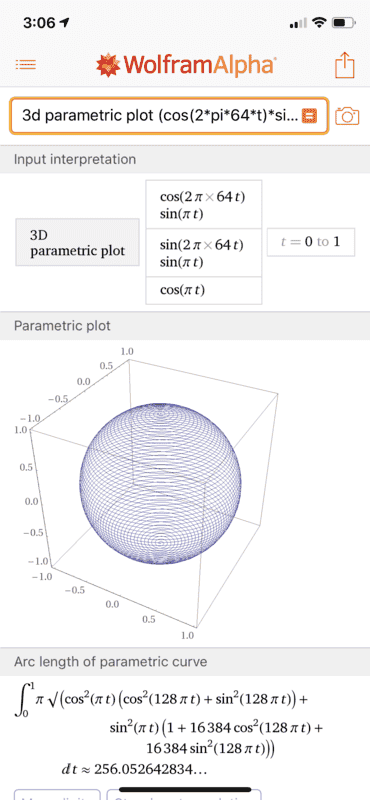metastable
Does this work as a very rough approximation:

I take a standard desk globe with its half arch that normally shows the offset of the Earth's rotation from the plane of the ecliptic.

I motorize the globe to turn at constant 2*pi rad sec.

I attach a motorized gantry to the arch with a marker contacting the globe. The marker can traverse the arch at constant angular speed from pole to pole.

The motorized gantry pen crosses over the north pole at constant angular speed 0.001rad/sec while the globe turns at constant 1 rad/sec angular speed until the marker gantry eventually crosses the south pole at constant angular speed completing the spiral.

The vector is referenced by referencing a number between one and zero. To calculate this number choose a point along the spiral and calculate the length along the spiral from the north pole to the chosen point, and divide this length by the total length along the spiral from the north pole to the south pole. The vector is given by a ray from the center of the sphere through the chosen point on the spiral. Position is given by vector + distance, for example: Vector: 0.456757263463... Distance: 15.3654... meters

metastableThank you so much for making this plot! It looks amazing.

I notice in the setup parameters, besides "2" and "pi" the only other number appears to be "64..." then I see the arc length appears to be "256.052642834..."

256.052642834... is very close to 64*2^2 -- but not quite... any idea what factors determine the divergence?

suremarc
256.052642834 is very close to 64*2^2 -- but not quite... any idea what factors determine the divergence?
If you work out the arc length, asymptotically one has that ##S\sim 4n## as ##n\rightarrow\infty##, where ##n## is the number of cycles. I digress.

In any case, I think you are missing the point here. You said you want to represent a vector to arbitrary precision with one number. Any spiral you construct will inherently have limited precision, because it does not cover the whole sphere.

Mathematically speaking, you could represent a vector via a sequence of increasingly accurate approximations (a Cauchy sequence). This is akin to certain constructions of the real numbers, so one could say that these constructions represent real numbers to “arbitrary precision”. The only problem is, you need an infinite number (a sequence) of approximations to represent something in this way.

So yes, you could use the spiral construction to make an “arbitrary precision” representation of 3d vectors, where each approximation labels a vector with a distance and a position on the spiral, and these approximations converge to some vector. But you’d need an infinite list of distances and positions.

metastable
I made an animation displaying what it looks like as the "orbits" increase: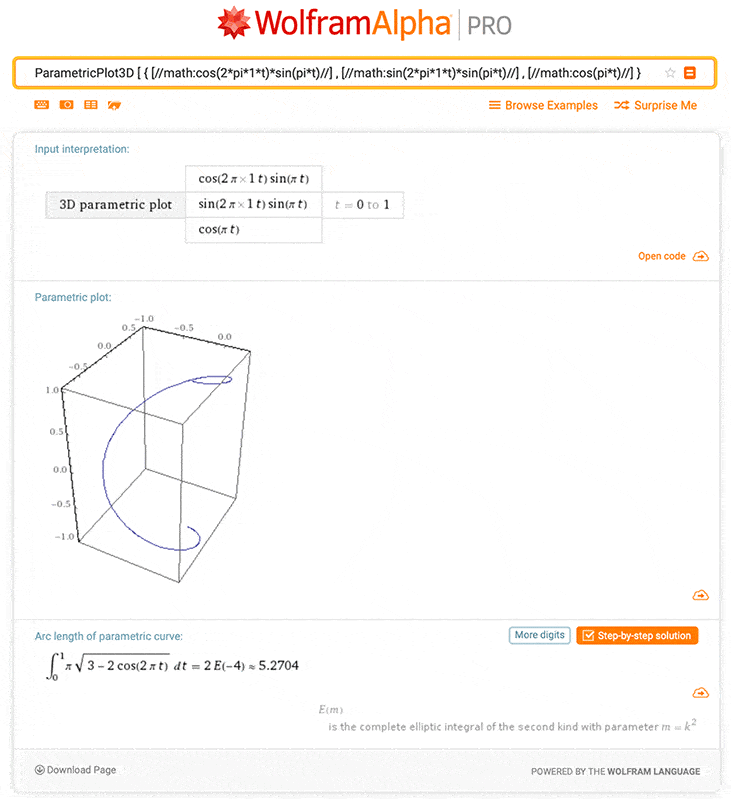metastable
Assuming your spiral is based on a model of a perfectly smooth sphere, you'd still need a reference to elevation.

Suppose instead of relying on a "reference to elevation" in the manner which I previously described (ie the center of the "sphere"), which consisted of a point that does not lie along the spiral, instead I rely only on points along the spiral itself to point to the vector. I make a straight line from point 0% to point 100%. Next I bisect line 0%-100% creating a point A. The vector is created first by a line from point A, through the referenced spiral point B to point C, a point in space. Points in space are referenced as Vector: 87.35775...% Distance: 686,739,974.97969...km Does this new convention "avoid reference to elevation?"

metastable
I made a bit of goof-up previously so this post is to make a slight correction:

Suppose to create a spiral on the surface of a sphere I do the following:

Where sphere radius = 1, I place both points A and B at the same location on a rotating sphere surface. The sphere rotates at 2*pi radians per second.

Point A is fixed on the sphere at maximum distance from the rotation poles while point B is free to traverse the surface only with constant surface distance/time directly towards one of the rotation poles, but otherwise point B co-rotates with the same frequency 2*pi rad/sec frequency as point A from a non-rotating perspective.

The spiral would be drawn from the path through space taken by point B (from a non-rotating perspective) where path length AB including the sphere's 2*pi rad/sec rotation = 2 *(((((1+sqrt(5))/2)^2)/5)*6) = 2 * 3.141640... = 6.28328... which is slightly greater than 2*pi

If I look at the co-rotating distance along the surface after 1 second between points AB, this distance gives me a surface velocity for moving point B relative to point fixed A from the rotating perspective.
Assuming point B continues moving along the surface at a constant surface velocity away from point A, then point B forms the rest of the half-sphere spiral from the non-rotating perspective. Point C forms the other half-sphere spiral completing the whole sphere spiral by repeating the operation with the sphere rotating with the same angular frequency in the opposite direction, with point C having freedom of movement only towards the opposite pole as point B.

My questions are:

-would this be a valid method of describing the sphere-spiral?

-will the use of closer and closer approximations to pi which are greater than pi yield more and more accurate vector definitions when referencing points along the spiral in relation to the center of the spiral?

After looking at the spiral a bit more I began having nagging doubts that maybe I'd gotten it wrong... Perhaps after 1 rotation, point B actually takes a SHORTER path through space than point A and 2pi. After looking at the problem a bit more, I make 2 observations:

-Point B initially covers more distance per unit time than point A
-But after 1 complete sphere rotation, point A has actually covered more distance than point B, so technically parts of what I had written were wrong

-Both of the following plots show different portions of point B's path along the same curve.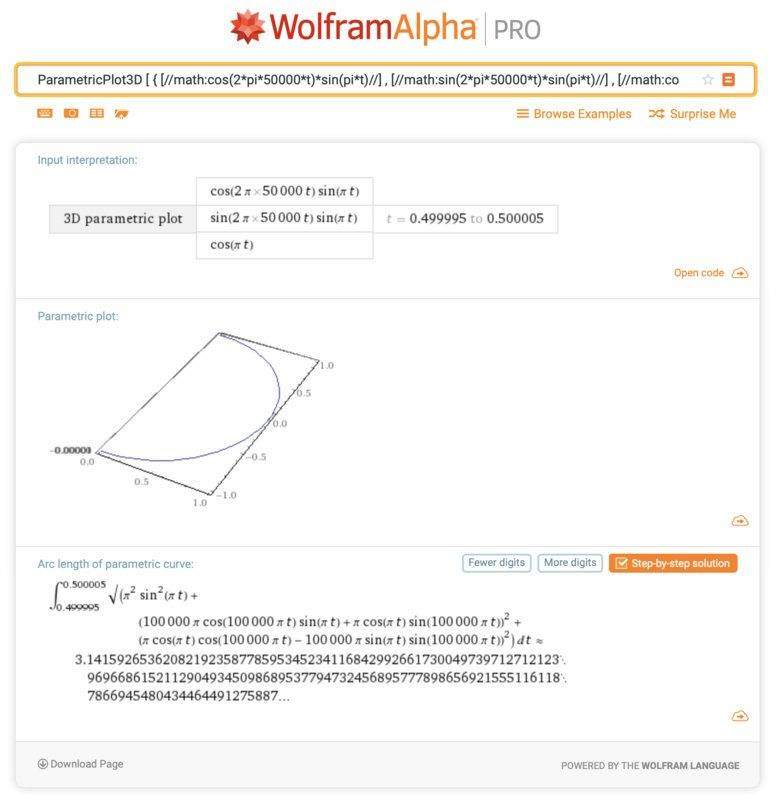^This plot's half orbit arc length is slightly larger than pi, and represents point B initially traveling faster than point A.^This plot's half orbit arc length is slightly smaller than pi, and represents point B covering less distance than point A when measured after 1 sphere rotation

Last edited:
metastable
^This plot's half orbit arc length is slightly smaller than pi, and represents point B covering less distance than point A when measured after 1 sphere rotation

Well after even further investigation it turns out the situation is even more complicated... if point B's path length after one sphere rotation is close enough to 2pi but also greater than 2pi, then point B can travel a distance greater than 2pi and path length A after one complete sphere rotation...

2 *(((((1+sqrt(5))/2)^2)/5)*6)

^The above equation is not close enough to 2pi to achieve length B after one rotation >2pi

However, this arc length describing point B's arc length after 1 sphere rotation is greater than 2 pi...

ParametricPlot3D [ { [//math:cos(2*pi*500000000*t)*sin(pi*t)//] , [//math:sin(2*pi*500000000*t)*sin(pi*t)//] , [//math:cos(pi*t)//] } , { t , [//number:0.5//] , [//number:0.5000000020//] } ]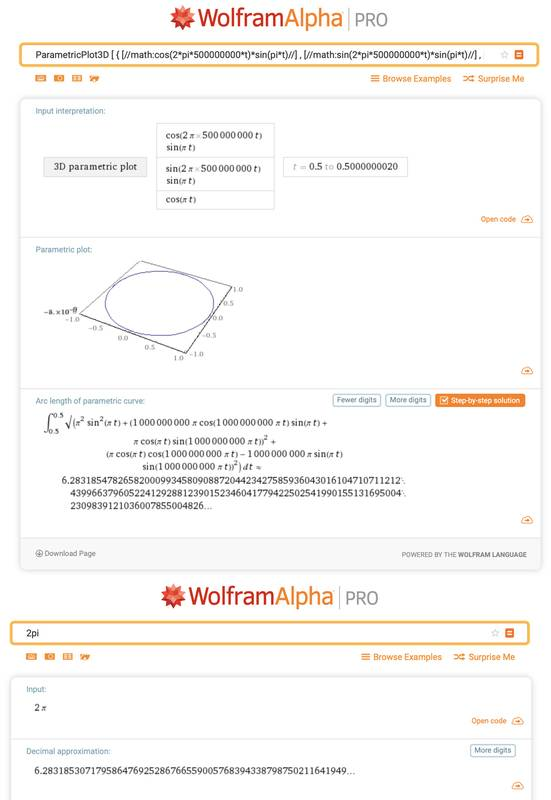In other words, if points A and B are in a "race through space" to 2pi distance traveled starting from the equator of a rotating 1 radius sphere, & point A can't change its surface coordinates on the sphere & point B can only move at constant surface velocity along the co-rotating surface of the sphere directly towards one of the poles, then point B can "win a race" to 2pi distance traveled through space after one sphere rotation, but only if point B's constant surface velocity toward the pole is low enough...

Last edited:
metastable
I want to calculate the limit, which is greater than 2pi, of how far through space point B can travel after exactly one sphere rotation, by only heading north from the equator with constant surface velocity? Is this limit certainly irrational? And if this sphere were approximately the size scale of the earth, what's the fastest surface velocity or surface angular speed in rad/sec I can roll "north" to "beat" someone who's just resting on the equator to 2pi arc length through space assuming 1 = approximated Earth radius and the sphere rotates 2pi rad/sec?

Last edited:
Protea Grandiceps
Does this new convention "avoid reference to elevation?"
Not sure if you still find this relevant, but yes, it would define elevation. It would complete reference to a 3rd dimension after (1st-D) in terms of no. of "orbits" and (2nd-D) in terms of % of distance from NP to SP since I'm assuming that we're still in a sphere of unitary size (r=1). In order to express 3D entirely within the realm of "orbits" it might be a good idea to express the 3rd-D reference in terms of total distance along the "orbits'" path from NP to SP since we would effectively be defining the radius of the sphere beyond r=1.